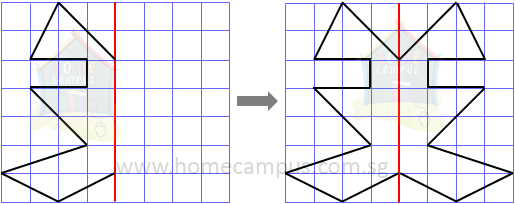## Symmetric Shapes, Figures and Patterns

Practice Unlimited Questions

#### 1. What are symmetrical shapes?

If a figure can be folded or divided into half so that the two halves match exactly then such a figure is called a symmetric figure.

The figures below are symmetric.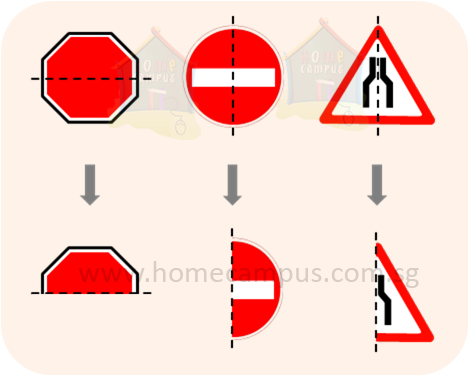The dotted line in each of the symmetric figures above that divides the figure into two equal halves is called the line of symmetry.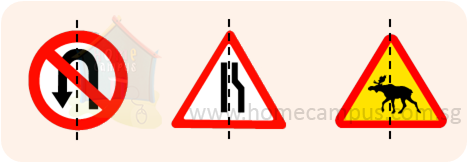In the figures above, there are no lines of symmetry that divide each of the figures into two equal halves. Therefore, these figures are not symmetric.

#### 2. Which ones of the following dotted lines are lines of symmetry of the oval?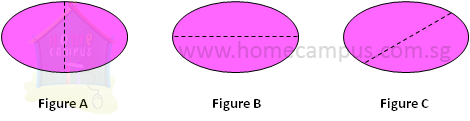Figure A:
Let's fold the oval along the dotted line.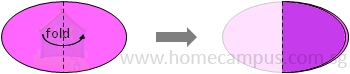The dotted line divides the oval into equal halves that match exactly. Hence, it is a line of symmetry.

Figure B:
Let's fold the oval along the dotted line.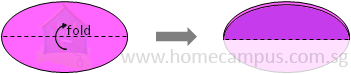The dotted line divides the oval into equal halves that match exactly. Hence, it is a line of symmetry.

Figure C:
Let's fold the oval along the dotted line.The dotted line does not divide the oval into equal halves that match exactly. Hence, it is not a line of symmetry.

#### 3. Making symmetric figures and patterns - Example 1

Step 1: Take a piece of paper and fold it.Step 2: Cut out any figure which starts and ends on the fold line such as the one shown below.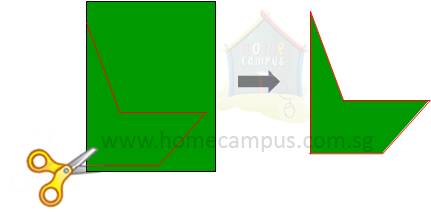Step 3: Unfold the figure. You have a symmetric figure where the fold line is the line of symmetry.#### 4. Making symmetric figures and patterns - Example 2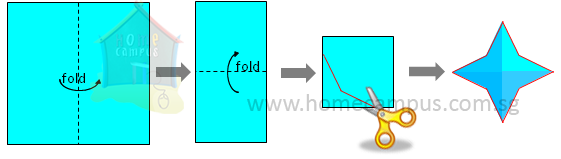#### 5. Making symmetric figures and patterns - Example 3

Each of the following figures below shows half of a symmetric pattern on a square grid. Complete each symmetric pattern with the red line as the line of symmetry.

a)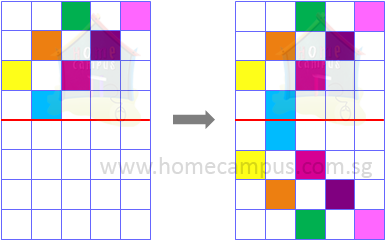b)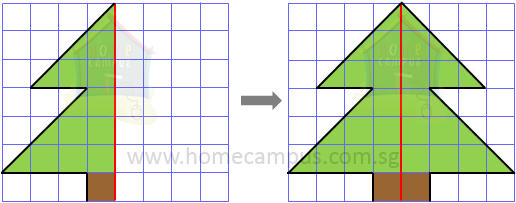c)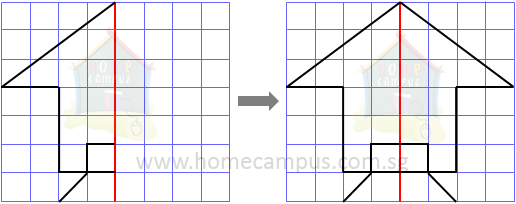d)